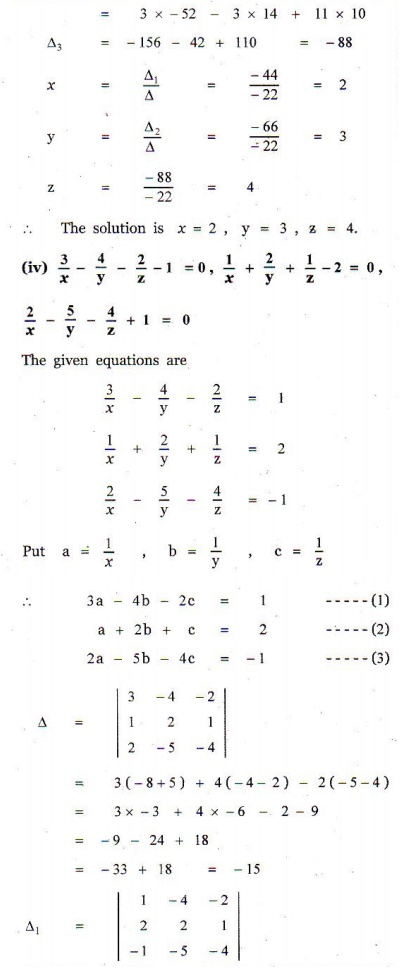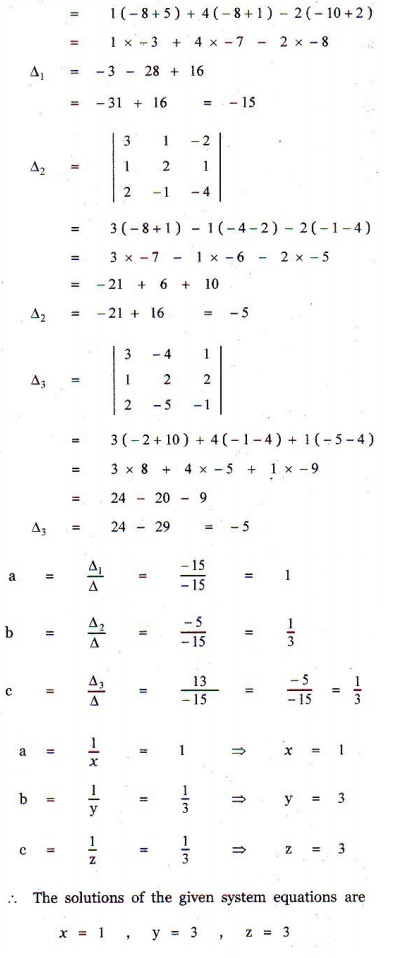Home | | Maths 12th Std | Exercise 1.4: Matrices: Cramerâ€™s Rule

# Exercise 1.4: Matrices: Cramerâ€™s Rule

Maths Book back answers and solution for Exercise questions - Solve the following systems of linear equations by Cramerâ€™s rule

EXERCISE 1.4

1. Solve the following systems of linear equations by Cramerâ€™s rule:

(i) 5x - 2 y +16 = 0, x + 3y - 7 = 02. In a competitive examination, one mark is awarded for every correct answer while ÂĽ mark is deducted for every wrong answer. A student answered 100 questions and got 80 marks. How many questions did he answer correctly ? (Use Cramerâ€™s rule to solve the problem).3. A chemist has one solution which is 50% acid and another solution which is 25% acid. How much each should be mixed to make 10 litres of a 40% acid solution ? (Use Cramerâ€™s rule to solve the problem).4. A fish tank can be filled in 10 minutes using both pumps A and B simultaneously. However, pump B can pump water in or out at the same rate. If pump B is inadvertently run in reverse, then the tank will be filled in 30 minutes. How long would it take each pump to fill the tank by itself ? (Use Cramerâ€™s rule to solve the problem).5. A family of 3 people went out for dinner in a restaurant. The cost of two dosai, three idlies and two vadais is â‚ą 150. The cost of the two dosai, two idlies and four vadais is â‚ą 200. The cost of five dosai, four idlies and two vadais is â‚ą 250. The family has â‚ą 350 in hand and they ate 3 dosai and six idlies and six vadais. Will they be able to manage to pay the bill within the amount they had ?1. (i) x = -2, y = 3

(ii) x = 1/2 , y = 3

(iii) x = 2, y = 3, z = 4

(iv) x = 1, y = 3, z = 3

2. 84

3. 50% acid is 6 litres, 25% acid is 4 litres

4. Pump A : 15 minutes, Pump B : 30 minutes

5. â‚ą 30/-, â‚ą 10/-, â‚ą 30/-, yes

Tags : Problem Questions with Answer, Solution , 12th Mathematics : UNIT 1 : Applications of Matrices and Determinants
Study Material, Lecturing Notes, Assignment, Reference, Wiki description explanation, brief detail
12th Mathematics : UNIT 1 : Applications of Matrices and Determinants : Exercise 1.4: Matrices: Cramerâ€™s Rule | Problem Questions with Answer, Solution